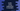# Kotlin tutorial : String in Kotlin with examples### What is a string :

Similar to Java, strings are a series of characters in Kotlin. String type is used for string variables. We can create one string variable similar to other. For example :

``````fun main(args: Array) {
val helloWorld = "Hello World !!"
val helloWorld2 : String = "Hello World !!"
}``````

Both helloWorld and helloWorld2 are string variables.

### Accessing character and iterating through a string :

In Kotlin, we can access any character of a string using its index, same as Java. The index starts from 0. For example :

``````fun main(args: Array) {
val helloWorld = "Hello World !!"

print(helloWorld)
print(helloWorld)
print(helloWorld)
}``````

It will print :

``HoW``

To iterate through a string, we can use one for-loop. We can iterate a string character by character in kotlin.

``````fun main(args: Array) {
val helloWorld = "Hello World !!"

for(c in helloWorld){
print(c)
}
}``````

The above program will print the complete string. You can try println instead of print to print all characters on different lines.

### Concatenate two strings :

We can concatenate two strings using the plus + operator like Java. The string is immutable in Kotlin, so it will create one new string variable on concatenation. For example :

``````fun main(args: Array) {
val firstPart = "Hello"
val secondPart = " World !!"
var helloWorld = firstPart + secondPart;

println(helloWorld)
}``````

It will print Hello World !! as the output.

### Types of String literals in Kotlin:

Kotlin has two types of string literals: Escaped string and raw string. An escaped string contains one escaped character in it. For example, the below string contains tab (\t) in it.

``````fun main(args: Array) {
val myString = "Hello\tWorld!!"
println(myString)
}``````

Supported escaped characters in Kotlin are : \t, \b, \n, \r, ’, ”, \ and \$. raw string is a string defined inside a triple quote """. It doesn’t contain any escaped character and we can even use newline characters in it. For example :

``````fun main(args: Array) {
val myString = """This is the first line
This is the second line
This is the third line
And fourth line
"""

println(myString)
}``````

It will print the following output :

``````This is the first line
This is the second line
This is the third line
And fourth line``````

To remove these spaces, we can use trimMargin() function. ’|’ is used as margin prefix. Check the below example :

``````fun main(args: Array) {
val myString = """This is the first line
|This is the second line
|This is the third line
|And fourth line
"""

println(myString.trimMargin())
}``````

Output :

``````This is the first line
This is the second line
This is the third line
And fourth line``````

We can also use a different character for margin prefix. For example, if ’<’ is a margin prefix, we can use trimMargin(”<“) to trim the whitespaces.

### String templates :

In kotlin string, we can use expressions to print including the string. It is called a template expression. It should start with a dollar sign (\$). Using it, we can print other values :

``````fun main(args: Array) {
val count = 10

print("value of count is \$count")
}``````

Output :

``value of count is 10``

Similarly, using an expression :

``````fun main(args: Array) {
val count = 10

print("value of count is \${if (count == 10) "equal to 10" else "not equal to 10"}")
}``````

It will print :

``value of count is equal to 10``